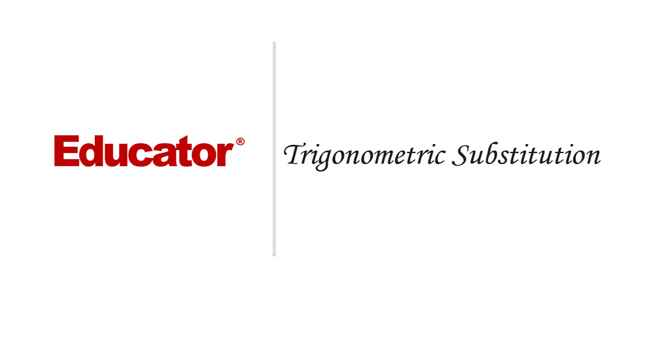Professor Murray

Trigonometric Substitutions

Slide Duration:

Integration by Parts

24m 52s

Intro
0:00
Important Equation
0:07
Where It Comes From (Product Rule)
0:20
Why Use It?
0:35
Lecture Example 1
1:24
Lecture Example 2
3:30
Shortcut: Tabular Integration
7:34
Example
7:52
Lecture Example 3
10:00
Mnemonic: LIATE
14:44
Ln, Inverse, Algebra, Trigonometry, e
15:38
-1
-2
Integration of Trigonometric Functions

25m 30s

Intro
0:00
Important Equation
0:07
Powers (Odd and Even)
0:19
What To Do
1:03
Lecture Example 1
1:37
Lecture Example 2
3:12
Half-Angle Formulas
6:16
Both Powers Even
6:31
Lecture Example 3
7:06
Lecture Example 4
10:59
-1
-2
Trigonometric Substitutions

30m 9s

Intro
0:00
Important Equations
0:06
How They Work
0:35
Example
1:45
Remember: du and dx
2:50
Lecture Example 1
3:43
Lecture Example 2
10:01
Lecture Example 3
12:04
-1
-2
Partial Fractions

41m 22s

Intro
0:00
Overview
0:07
Why Use It?
0:18
Lecture Example 1
1:21
Lecture Example 2
6:52
Lecture Example 3
13:28
-1
-2
Integration Tables

20m

Intro
0:00
Using Tables
0:09
Match Exactly
0:32
Lecture Example 1
1:16
Lecture Example 2
5:28
Lecture Example 3
8:51
-1
-2
Trapezoidal Rule, Midpoint Rule, Left/Right Endpoint Rule

22m 36s

Intro
0:00
Trapezoidal Rule
0:13
Graphical Representation
0:20
How They Work
1:08
Formula
1:47
Why a Trapezoid?
2:53
Lecture Example 1
5:10
Midpoint Rule
8:23
Why Midpoints?
8:56
Formula
9:37
Lecture Example 2
11:22
Left/Right Endpoint Rule
13:54
Left Endpoint
14:08
Right Endpoint
14:39
Lecture Example 3
15:32
-1
-2
Simpson's Rule

21m 8s

Intro
0:00
Important Equation
0:03
Estimating Area
0:28
Difference from Previous Methods
0:50
General Principle
1:09
Lecture Example 1
3:49
Lecture Example 2
6:32
Lecture Example 3
9:07
-1
-2
Improper Integration

44m 18s

Intro
0:00
Horizontal and Vertical Asymptotes
0:04
Example: Horizontal
0:16
Formal Notation
0:37
Example: Vertical
1:58
Formal Notation
2:29
Lecture Example 1
5:01
Lecture Example 2
7:41
Lecture Example 3
11:32
Lecture Example 4
15:49
Formulas to Remember
18:26
Improper Integrals
18:36
Lecture Example 5
21:34
Lecture Example 6 (Hidden Discontinuities)
26:51
-1
-2
Section 2: Applications of Integrals, part 2
Arclength

23m 20s

Intro
0:00
Important Equation
0:04
Why It Works
0:49
Common Mistake
1:21
Lecture Example 1
2:14
Lecture Example 2
6:26
Lecture Example 3
10:49
-1
-2
Surface Area of Revolution

28m 53s

Intro
0:00
Important Equation
0:05
Surface Area
0:38
Relation to Arclength
1:11
Lecture Example 1
1:46
Lecture Example 2
4:29
Lecture Example 3
9:34
-1
-2
Hydrostatic Pressure

24m 37s

Intro
0:00
Important Equation
0:09
Main Idea
0:12
Different Forces
0:45
Weight Density Constant
1:10
Variables (Depth and Width)
2:21
Lecture Example 1
3:28
-1
-2
Center of Mass

25m 39s

Intro
0:00
Important Equation
0:07
Main Idea
0:25
Centroid
1:00
Area
1:28
Lecture Example 1
1:44
Lecture Example 2
6:13
Lecture Example 3
10:04
-1
-2
Section 3: Parametric Functions
Parametric Curves

22m 26s

Intro
0:00
Important Equations
0:05
Slope of Tangent Line
0:30
Arc length
1:03
Lecture Example 1
1:40
Lecture Example 2
4:23
Lecture Example 3
8:38
-1
-2
Polar Coordinates

30m 59s

Intro
0:00
Important Equations
0:05
Polar Coordinates in Calculus
0:42
Area
0:58
Arc length
1:41
Lecture Example 1
2:14
Lecture Example 2
4:12
Lecture Example 3
10:06
-1
-2
Section 4: Sequences and Series
Sequences

31m 13s

Intro
0:00
Definition and Theorem
0:05
Monotonically Increasing
0:25
Monotonically Decreasing
0:40
Monotonic
0:48
Bounded
1:00
Theorem
1:11
Lecture Example 1
1:31
Lecture Example 2
11:06
Lecture Example 3
14:03
-1
-2
Series

31m 46s

Intro
0:00
Important Definitions
0:05
Sigma Notation
0:13
Sequence of Partial Sums
0:30
Converging to a Limit
1:49
Diverging to Infinite
2:20
Geometric Series
2:40
Common Ratio
2:47
Sum of a Geometric Series
3:09
Test for Divergence
5:11
Not for Convergence
6:06
Lecture Example 1
8:32
Lecture Example 2
10:25
Lecture Example 3
16:26
-1
-2
Integral Test

23m 26s

Intro
0:00
Important Theorem and Definition
0:05
Three Conditions
0:25
Converging and Diverging
0:51
P-Series
1:11
Lecture Example 1
2:19
Lecture Example 2
5:08
Lecture Example 3
6:38
-1
-2
Comparison Test

22m 44s

Intro
0:00
Important Tests
0:01
Comparison Test
0:22
Limit Comparison Test
1:05
Lecture Example 1
1:44
Lecture Example 2
3:52
Lecture Example 3
6:01
Lecture Example 4
10:04
-1
-2
Alternating Series

25m 26s

Intro
0:00
Main Theorems
0:05
Alternation Series Test (Leibniz)
0:11
How It Works
0:26
Two Conditions
0:46
Never Use for Divergence
1:12
Estimates of Sums
1:50
Lecture Example 1
3:19
Lecture Example 2
4:46
Lecture Example 3
6:28
-1
-2
Ratio Test and Root Test

33m 27s

Intro
0:00
Theorems and Definitions
0:06
Two Common Questions
0:17
Absolutely Convergent
0:45
Conditionally Convergent
1:18
Divergent
1:51
Missing Case
2:02
Ratio Test
3:07
Root Test
4:45
Lecture Example 1
5:46
Lecture Example 2
9:23
Lecture Example 3
13:13
-1
-2
Power Series

38m 36s

Intro
0:00
Main Definitions and Pattern
0:07
What Is The Point
0:22
0:45
Interval of Convergence
2:42
Lecture Example 1
3:24
Lecture Example 2
10:55
Lecture Example 3
14:44
-1
-2
Section 5: Taylor and Maclaurin Series
Taylor Series and Maclaurin Series

30m 18s

Intro
0:00
Taylor and Maclaurin Series
0:08
Taylor Series
0:12
Maclaurin Series
0:59
Taylor Polynomial
1:20
Lecture Example 1
2:35
Lecture Example 2
6:51
Lecture Example 3
11:38
Lecture Example 4
17:29
-1
-2
Taylor Polynomial Applications

50m 50s

Intro
0:00
Main Formulas
0:06
Alternating Series Error Bound
0:28
Taylor's Remainder Theorem
1:18
Lecture Example 1
3:09
Lecture Example 2
9:08
Lecture Example 3
17:35
-1
-2
Bookmark & Share Embed

## Copy & Paste this embed code into your website’s HTML

Please ensure that your website editor is in text mode when you paste the code.
(In Wordpress, the mode button is on the top right corner.)
×
• - Allow users to view the embedded video in full-size.
Since this lesson is not free, only the preview will appear on your website.

• ## Related Books1 answer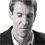Last reply by: Dr. William MurrayMon Feb 4, 2019 2:25 PMPost by Hessa on February 1, 2019how to solve this question using trig subthe integral of x(1-x^4) ^1/2?1 answerLast reply by: Dr. William MurrayMon Jan 28, 2019 11:43 AMPost by Hessa on January 27, 2019I did not understand example 31 answerLast reply by: Dr. William MurrayThu Aug 4, 2016 6:01 PMPost by Peter Ke on July 27, 2016For example 3, you let u = 3tan(theta). Where did that idea come from and why?1 answerLast reply by: Dr. William MurraySat Feb 27, 2016 10:36 AMPost by Mohsin Alibrahim on February 25, 2016Dr Murray, In example 3, how come du = [3sec^2(x)]  while u = 9sec^2(x) ?Thanks for the wonderful lecture3 answersLast reply by: Dr. William MurrayFri Apr 10, 2015 1:07 PMPost by Luvivia Chang on April 7, 2015Hello Dr. Murray, I have a question about exemple 1i think the square root of (1-sin^2(theta))=the square root of cos^2(theta)= the "absolute value of cos(theta)" instead of just cos (theta)Am I correct? Thanks2 answersLast reply by: Dr. William MurrayThu Feb 19, 2015 3:48 PMPost by Thadeus McNamara on February 18, 2015Dr. Murray, I am taking the Calc BC Exam. Are there any lectures in this College Calculus II playlist that I can skip because they are not a part of the exam? Thanks2 answersLast reply by: Dr. William MurrayThu Feb 19, 2015 3:48 PMPost by Thadeus McNamara on February 18, 2015@4:51, isn't the integral of 2/3sintheta equal to -2/3costheta? You put the integral as 2/3costheta.3 answersLast reply by: Dr. William MurrayWed Aug 27, 2014 9:46 AMPost by brody wagerson on August 24, 2014I believe there is an error in lecture example 4, on the last step to simplify the answer you cannot divide x^2-6x+9 by 9 to get x^2-6x, because they are addition and not multiplication. You must multiply out what is under the square root sign to get 9-(x^2-6x+9) the 9's will then cancel and give you 6x-x^2 under the square root just like in the beginning of the question, but without taking out an extra 1/3 to divide. Sorry if my explanation does not make sense I wrote this quickly. but i believe the correct answer to example 4 if I am correct will be 9/2[arcsin(x-3/3)+(x-3/3)*sqrt(6x-x^2)]+C. No stranger to criticism though so let me know if I made a mistake and professor Murray is right.1 answerLast reply by: Dr. William MurrayTue Aug 5, 2014 3:18 PMPost by Pedro Valdericeda on July 21, 2014Thank you Prof. Murray. You are really good.1 answerLast reply by: Dr. William MurrayTue Jun 17, 2014 12:03 PMPost by ahmed ahmed on June 9, 2014thank you professor! you are awesome!1 answerLast reply by: Dr. William MurrayMon Mar 17, 2014 1:13 PMPost by Peidong, He on March 16, 2014WANNA SAY THANK YOU!1 answerLast reply by: Dr. William MurrayFri Aug 23, 2013 11:52 AMPost by Charles Zhou on August 21, 2013This is absolutely a nice video! It does help me a lot. I just have a question. I'm a high school student and I wonder is there any difference or relationship between AP calculus BC and this College Calculus Level II1 answerLast reply by: Dr. William MurrayThu Dec 6, 2012 5:40 PMPost by Samuel Bass on December 6, 2012In problem #1 in the solution. You converted cos(theta) = squar(1-sin^2(theta)) yes this would be correct if the cos(theta) was squared e.g. cos^2(theta)but in this case the cos(theta) is not raised to the power 2.... then you can't use the pythagoream Identitie as you did in this case..... Im I correct? Cause I can't see this specific step you did possible. Please Explain.1 answerLast reply by: Dr. William MurrayMon Oct 22, 2012 1:48 PMPost by Keisha Baxter on October 21, 2012He factored incorrectly in Lecture example 1... in about the third line when he factors out the 4 from sqrt(4 - 4 sin^2 x) he puts 2 sqrt (1 - sin^2 x). So all of the coefficients following that is incorrect1 answerLast reply by: Dr. William MurrayThu Apr 18, 2013 12:24 PMPost by SOUFIANE LAMOUNI on May 16, 2012you are a great Professor , thank you1 answerLast reply by: Dr. William MurrayThu Apr 18, 2013 12:23 PMPost by Kumar Sandrasegaran on February 26, 2012Very very interesting. Makes difficult calculations look simple1 answerLast reply by: Dr. William MurrayThu Apr 18, 2013 12:20 PMPost by Real Schiran on November 22, 2011Very Nice, Thanks...........1 answerLast reply by: Dr. William MurrayThu Apr 18, 2013 12:19 PMPost by Jaspreet Singh on February 21, 2010this is so much more helpful than school! thanks for being so clear1 answerLast reply by: Dr. William MurrayThu Apr 18, 2013 12:19 PMPost by lynette stevenson on February 17, 2010excellent lecture

### Trigonometric Substitutions

Main formula:

1. For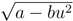, substitute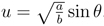.

2. For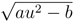, substitute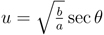.

3. For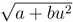, substitute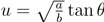.

Hints and tips:

• Remember to make the substitution as well as the main substitution.

• You can sometimes use trigonometric substitution even when you don’t have a square root. However, in these cases, you should only be using the tangent substitution. If you get one of the other ones, then you should have been able to factor the algebraic expression, and trigonometric substitution was not necessary.

• If you have a linear term (x or u, as opposed to x² or u²), complete the square and make a substitution to eliminate the linear term before you make the trigonometric substitution.

• If the coefficient of the quadratic term (x²) is negative, factor the negative out of all terms before completing the square.

• After you make the trigonometric substitution, it becomes a trigonometric integral that you can handle using the techniques in the previous section.

### Trigonometric Substitutions

Lecture Slides are screen-captured images of important points in the lecture. Students can download and print out these lecture slide images to do practice problems as well as take notes while watching the lecture.

• Intro 0:00
• Important Equations 0:06
• How They Work
• Example
• Remember: du and dx
• Lecture Example 1 3:43
• Lecture Example 2 10:01
• Lecture Example 3 12:04

### Transcription: Trigonometric Substitutions

This is educator.com.0000

Here are a couple more examples on trigonometric substitution.0003

We are going to start with the integral of 6x x2 dx.0006

We have a quadratic under the sqrt.0012

The problem is we do not have a constant - x2.0016

We have 6x - x2.0020

What we have to do is complete the square on that before we go ahead with our trigonometric substitution.0022

Because we do not have a constant yet.0030

I am going to write that as sqrt, I am going to factor out the negative sign first, and I will get - x2 - 6x,0031

And now I want to complete the square here.0043

So, I take the middle term, the 6x, and divide it by 2 and square it.0046

So -6/-2 is 3, you square that and you get 9, add on 9.0051

Now to pay for that, well I added 9, but that was inside the negative sign right here.0059

So, what I really did there was I subtracted 9,0069

So, to balance that out I have to add 9.0072

We get sqrt(-x2-6x+9)+9.0079

I will write that as sqrt(9) -, and the whole point of completing the square there is that this was (x-3)2.0090

The first thing I am going to do here is a little substitution.0102

Let u = (x-3), and we always have to substitute the du as well.0109

The du is dx there, so it is an easy substitution but we have to do it.0112

What we have here is the integral of sqrt(9-u2) du.0120

Now, this is something that looks like it is ready for a trig substitution.0131

We look at the trig substitution rules and we see that this one calls for a sin substitution,0135

Because it is 9 minus u2, the u is being subtracted.0142

So we are going to let u = 3sin(θ).0146

Again, I got this 3 because it is the square root of this nine.0154

du is going to be 3cos(θ) dθ.0160

The point of doing that is the sqrt(9-u2), well, u2 = 92θ.0171

If we pull a 9 out of the radical we get 3 × (1-sin2θ).0190

1-sin2θ is cos2θ, so this is just 3cos(θ).0199

The radical turns into 3cosθ, the du turns into 3cos(θ) dθ.0204

This whole integral turns into 9cos2θ dθ.0219

Again, we have a trigonometric integral in the trigonometric integral lecture, that when you see even powers of cosine,0228

you use the half-angle formula.0233

This is 9 × the integral of 1/2.0237

I will just pull the 1/2 outside, 9/2, times the integral of 1 + cos(2θ) dθ.0243

So, that is 9/2, if you integrate 1 you get θ + integral of cos is sine,0256

But because of that 2, we have to have a 1/2 there, and this was all being multiplied by the 9/2.0272

We will still have a constant.0282

Again, we have sin(2θ).0285

We have seen this earlier and the way we want to resolve sin(2θ) is 2sin(θ) cos(θ).0290

So, I am going to keep going on the next page here.0301

Let us remember the important things are that u was 3sin(θ), and in turn u was (x-3).0307

9 - u2 was 3cos(θ).0316

We will be using the sin(2θ) and I am just going to copy this equation on the next page and keep going.0322

From the previous page we have 9/2 × θ + 1/2 sin(2θ) + C.0331

So, 9/2, how can we sort θ out?0347

Well remember that u was 3sinθ.0353

So, θ if you solve this, u/3 is sin(θ).0364

θ is arcsin(u/3).0372

The 1/2 sin(2θ), remember sin(2θ) is 2sin(θ) cos(θ).0378

So 1/2 sin(2θ) is sin(2θ) cos(θ).0390

So we have 9/2 arcsin(u/3), sin(θ) is u/3.0403

Now the cos(θ), what we remember from before is that cos(θ).0416

Actually we cannot see it here, so maybe I will work it out again very quickly.0424

Cos(θ) is the sqrt(1 - sin2(θ)), which is sqrt(1) -, sin(θ) is u/3, so we know sin(θ) is u2/90430

Cos(θ) is sqrt(1 - u2/9).0445

And, so, this turns into 9/2 arcsin, OK u/3 it is time to convert that back into, remember u is x-3.0456

So x-3/3 + now we have u/3 again.0470

That is x-3/3 again.0475

Now 1 - u2/9 is, 1 - u2 is (x-3)2/9.0483

I am just going to try to simplify this radical because I think you will recognize it after I simplify it.0498

That is 1 - (x-3)2, is x2 - 6x + 9/9.0504

We are going to write 1 as 9/9.0518

The 9/9's cancel, and we get 9/2 arcsin(x-3/3) + (x-3)/3.0524

This radical turns into over 9 - x2 + 6x.0538

If you pull that 9 out, we get a 1/3 6x-x2, which is the same radical we started with.0547

So, our final answer is 9/2 arcsin(x-3/3) + x-3/9 × sqrt(6x - x2) + C.0559

That one was a pretty long example.0581

The key to it was recognizing this initial radical in the integral.0589

Recognizing that we had to complete the square on that.0594

Then we had to do a trigonometric sin substitution on that.0600

So, those were sort of the two key theoretical steps there.0611

The rest was just keeping track of lots of substitutions.0617

X into u, and u into θ0618

And then keeping track of a lot of different constants that were percolating throughout the whole interval.0625

A very long and complicated example but the basic ideas were just completing the square and doing a basic substitution.0630

So I want to do one more example.0000

This is the integral of dx/sqrt(x2 + 1).0004

Remember, when you see x2 + 1, that is your sort of warning flag that you are going to need a tangent substitution.0009

Remember a minus tells you that you are going to use either a sin or a secant substitution,0018

And a plus tells us that we are going to use a tangent substitution.0025

So we are going to use x = tan(θ), and then dx = sec2(θ) dθ.0027

x2 + 1 = tan2(θ + 1), which by the trigonometric identity is sec2(θ).0043

And, that was the whole point of making the substitution, to invoke that trigonometric identity,0059

That we would get tan2 + 1 and could convert it into sec2(θ).0064

The sqrt(sec2(θ) is just sec(θ).0072

When we solve this integral, or rather make this substitution into this integral,0077

We have dx in the numerator, so that converts into sec2(θ) dθ.0087

In the denominator we have the sqrt(x2 + 1),0093

We already saw that that is sec(θ).0094

So, the secants, one of the secants cancels and we just get the integral of sec(θ) dθ.0100

That is the trigonometric integral that we learned in the trigonometric integral lecture.0113

You have to remember how to do it.0116

There is an old trick, where you multiply the top and bottom by sec(θ) tan(θ).0121

We covered that in the lecture on trigonometric integrals.0136

The answer for the integral of sec(θ) came out to be the ln(abs(sec(θ) + tan(θ).0140

That in turn, turns into, remember we have to substitute back into x.0153

sec(θ), I figure out what this right here, that is the sqrt of x2 + 1.0156

tan(θ) was our original substitution, that was x.0164

And, we have to put a constant on that.0168

Again, the key step there was recognizing that we had x2 + 1,0177

And recognizing that that would give us a tangent substitution.0181

It was also important to keep track of the dx,0188

And then to work everything into θ's, and then at the end convert everything back into x's.0190

So that is the end of the lecture on trigonometric substitutions.0195

This has been educator.com.0200

Welcome to educator.com, this is the lecture on trigonometric substitution.0000

There are three main equations that you have for trigonometric substitution.0006

The idea is that if you see any one of these three forms in an integral, then you can do what is called a trigonometric substitution to convert your integral into a trigonometric integral.0012

Then hopefully you can use some of the trigonometric techniques that we learned in the previous lecture to solve the integral.0028

Let me show you how they work.0035

If you see something like the sqrt(a-bu2).0038

Now here the a and the b are constants, and the u is the variable.0042

You are going to make the substitution u=sqrt(a/(bsin(θ)).0048

Why does that work?0051

Well, if you do that, then u2 is a/(bsin2θ),0053

And, so a-bu2 will be a - while bu2 will be asin2(θ)0063

That is a × (1-sin2(θ))0079

And 1-sin2(θ) of course is cos2(θ).0088

If you have the square root of that expression, then that will convert into cos(θ), and you will get an easier integral to deal with.0096

By the same idea, if you have au2-b, then you are going to have u=sqrt(b/(asec(θ)).0104

What you are trying to do there is take advantage of the trigonometric identity tan2(θ)+1=sec2(θ).0117

Sec2(θ)-1 = tan2(θ) so once you make this substitution you are going to end up with sec2(θ)-1 under the square root.0131

The last one you will have a+bu2=sqrt(a/(btan(θ))) and so again you are taking advantage of this trigonometric identity tan2(θ)+1.0144

You will end up with that under the square root, and that will convert into sec2(θ).0161

All of these take advantage of these trigonometric identities.0165

One thing that is important to remember when you are making these substitutions is whenever you substitute u equals something, or x equals something, you always have to substitute in dU or dX as well.0170

For example, if you substitute u=sqrt(a/bsin(θ)), then you also have to substitute dU, which would be, a and b are constants so that is just sqrt(a/bcos(θ)) dθ.0182

You always have to make the accompanying substitution for your dU or your dX.0204

As with all mathematical problems it is a little hard to understand when you are just looking at mathematical formulas in general, but we will move onto examples and you will see how these work.0211

The first example is the integral of 4 - 9x2 dx.0223

That example matches the first pattern that we saw before, which was the square root of a minus b u2.0229

The substitution that we learned for that is u equals the square root of a/b sin(θ).0237

Here, the a is 4, the b is 9, the x is taking the place of the u.0249

What we are going to substitute is x equals well the square root of a over b, is the square root of 4/9 sin(θ).0259

The square root of 4/9 is 2/3 sin(θ).0276

As we do that we also remember we have to substitute dX, which is going to be 2/3 cos(θ) dθ.0279

This is why it is really important to write the dX along with your integral.0291

It helps you remember that you also need to do the extra part of the substitution with the dX.0296

You have to convert that to the new variable as well.0302

So, then our integral becomes the integral of the square root of 4 minus, well 9x2 is 9, x2 is 4/9 sin2(θ) and dX is 2/3 cos(θ) dθ.0304

Just working inside the square root for a moment here, we get the square root of 4 minus 4 sin2(θ) and we can pull the 4 out of the square root.0334

We get 4 times the square root of 1 minus sin2θ.0343

That is 2 times 1 minus sin2(θ) is cos2(θ) so that will give us cos(θ).0352

Now, if we bring in the other elements of the integral, we get the integral.0360

I am going to collect all of the constants outside, so we have a 2, and a 2/3 that is 4/3 cos2(θ) because we have one cos here, and one cos here, dθ.0363

We learned how to integrate cos2(θ), you use the half angle formula.0380

This is 4/3 times the integral of 1/2 times one plus cos(2θ) dθ.0387

If we combine the 1/2 with the 4 we get 2/3 times now the integral of 1.0401

Remember we're integrating with respect to θ so that is θ plus the integral of cos(2θ).0412

Well the integral of cos is sin, but because of the 2 there, we have to put a 1/2 there.0421

This is now 2/3 and we want to convert this back to x's.0432

We have to solve these equations for θ in terms of x.0448

θ if we solve this equation in terms of x, we get 3/2 x equals sin(θ)0449

So θ is equal to arcsin(3/2 x)0465

Arcsin(3/2 x), that is the θ. Now 1/2 sin(2θ) to sort that out it helps to remember that sin(2θ) is 2 times sin(θ) times cos(θ)0478

1/2 2sin(θ) is sin(θ) times cos(v)0500

So, 2/3 arcsin(3/2 x)0515

Now, sin(θ) we said was 3/2 x.0525

Cos(θ) is the square root of 1 minus sin2(θ), so 1 minus 3/2 sin(θ) was x 9/4 x2.0532

Now we have converted everything back in terms of x.0554

The point here was that we started with a square root of a quadratic and we used our trigonometric substitution and we converted this integral into a trigonometric integral.0568

Then we learned in the other lecture how to convert trigonometric integrals, so we solved that integral in terms of θ.0588

Then we have to convert it back into terms of x.0592

So, let us try another example, this one is actually a little quicker.0598

We see the integral of dX over 1 + x20605

The key thing to remember here is that when you see the square root of 1 + x2, or even 1 + x2 without a root, you want to use the tangent substitution.0611

X equals the tan(θ)0629

The reason you do that is that x2 + 1 is tangent2(θ) + 1 but that is sec2(θ)0632

Let us make that substitution x equals tanθ.0642

Of course, every time you make a substitution you also have to make a substitution for dX.0650

dX is equal to sec2(θ) dθ0653

If we plug that into the integral, we get dX is sec2(θ) dθ.0663

1 + x2 is sec2(θ)0672

We kind of lucked out on this one because the sec2's cancel and we just get the integral of θ0680

This turns out to be a really easy one, that is just θ.0686

Theta, remember that x was tan(θ) we know that θ is arctan(x) + a constant.0692

The thing to remember about this example is that when you have a + there, you are going to go for a tangent substitution.0706

Whenever you have a minus, you are going to go for a secant substitution.0720

We are going to move to a more complicated example now.0727

dX/x2 + 8x + 25.0729

We have a more complicated expression in the denominator here.0737

What we are going to do is try to simplify it into something that admits a trigonometric substitution pretty easily.0738

What we are going to do is a little high school algebra on the denominator.0748

We are going to complete the square on that, so that is x20758

Remember, the way you complete the square is you take this 8 and you divide by 2 and you square it.0764

So 8 divide by 2 is 4 and 4 squared is 16, so we will write 16 there.0770

To make this a true equation, we have to add 9 there.0777

Then that is x + 4, quantity squared + 9.0780

We are going to use that for the integral.0791

What we are going to do is use that for the quick substitution.0795

U is equal to x + 4 and again we have to convert dU, but that is easy that is just dX.0797

The integral just converts into dU/u2 + 9.0811

Now, to finish that integral, well, we want to make a trigonometric substitution.0820

We are going to let u be 3tanθ.0829

The way I got 3tanθ was I looked at 9 and I took the square root of that and I got 3.0839

The point of doing that is that u2 + 9 will be 9tan2(θ)+9.0844

And, that is 9+tan2(θ)+1.0856

Which is 9 sec2(θ).0863

Of course with any substitution, you have to figure out what dU is.0867

Well if u is 3tan(θ) then dU is 3 sec2(θ) dθ.0871

We will plug all of that in, the dU was 3 sec2θ dθ.0881

The u2 + 9 converted into 9 sec2θ0890

The three and the 9 simplify down into 1/3 and now again, the secants cancel and we just have the integral of dθ.0900

That is 1/3 θ.0907

θ is something we can figure out from this equation over here.0914

θ if we solve this equation u/3=tanθ.0925

θ is arctan(u/3).0933

But we are not finished with that because we still have to convert back into terms of x.0938

That is 1/3 arctan, now u was (x+4), remember our original substitution there, so (x+4)/3 and now we add on a constant.0944

Now we are done.0960

OK, that is the end of the first instalment of trigonometric substitution.0966

This has been educator.com.0972

OR

### Start Learning Now

Our free lessons will get you started (Adobe Flash® required).